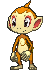Thread: Text Hex Decoder View Single Post6th September 2014, 05:42 PM
Kakarot1212
not that active anymore

Join Date: Dec 2013
Location: Philippines
Age: 21
Posts: 82Text Hex Decoder

UPDATE PEOPLE! {XD}

## What the heck is this tool?

This "poorly-made" tool converts Text values to Hex value, and vice-versa. And it is now written in VB.NET! After I decided to part ways with Java. For example if you want to change the game's battle messages,(see smeargles' tutorial HERE) you can use this tool to aid you in converting text values to hex values, and again, vice-versa. And now added support to standard hex!

If you're familiar with hex, converting is just a piece of cake for you. But what if not? Try to look at this:

Let's try to convert the string, "Hello world" for example. Now, how the heck can we convert this to hex? Take note that every letter has it's corresponding Hex equivalent. You can look at this list if you want:
Spoiler:

Pokemon MSG Hex Values
Spoiler:

00= (space)
01=À
02=Á
03=Â
04=Ç
05=È
06=É
07=Ê
08=Ë
09=Ì
0B=Î
0C=Ï
0D=Ò
0E=Ó
0F=Ô
10=
11=Ù
12=Ú
13=Û
14=Ñ
15=ß
16=à
17=á
19=ç
1A=è
1B=é
1C=ê
1D=ë
1E=ì
20=î
21=ï
22=ò
23=ó
24=ô
25=
26=ù
27=ú
28=û
29=ñ
2A=º
2B=ª
2D=&
2E=+
34=[Lv]
35==
36=;
51=¿
52=¡
53=[pk]
54=[mn]
55=[po]
56=[ké]
57=[bl]
58=[oc]
59=[k]
5A=Í
5B=%
5C=(
5D=)
68=â
6F=í
79=[U]
7A=[D]
7B=[L]
7C=[R]
85=<
86=>
A1=0
A2=1
A3=2
A4=3
A5=4
A6=5
A7=6
A8=7
A9=8
AA=9
AB=!
AC=?
AE=-
AF=·
B0=[.]
B1=[""]
B2=""
B3=[']
B4='
B5=[m]
B6=[f]
B7=[\$]
B8=,
B9=[x]
BA=/
BB=A
BC=B
BD=C
BE=D
BF=E
C0=F
C1=G
C2=H
C3=I
C4=J
C5=K
C6=L
C7=M
C8=N
C9=O
CA=P
CB=Q
CC=R
CD=S
CE=T
CF=U
D0=V
D1=W
D2=X
D3=Y
D4=Z
D5=a
D6=b
D7=c
D8=d
D9=e
DA=f
DB=g
DC=h
DD=i
DE=j
DF=k
E0=l
E1=m
E2=n
E3=o
E4=p
E5=q
E6=r
E7=s
E8=t
E9=u
EA=v
EB=w
EC=x
ED=y
EE=z
EF=[>]
F0=:
F1=Ä
F2=Ö
F3=Ü
F4=ä
F5=ö
F6=ü
F7=[u]
F8=[d]
F9=[l]

Standard Hex Values
Spoiler:

20=(space)
61=a
62=b
63=c
64=d
65=e
66=f
67=g
68=h
69=i
6a=j
6b=k
6c=l
6d=m
6e=n
6f=o
70=p
71=q
72=r
73=s
74=t
75=u
76=v
77=w
78=x
79=y
7a=z
41=A
42=B
43=C
44=D
45=E
46=F
47=G
48=H
49=I
4a=J
4b=K
4c=L
4d=M
4e=N
4f=O
50=P
51=Q
52=R
53=S
54=T
55=U
56=V
57=W
58=X
59=Y
5a=Z
7e=~
60=`
21=!
[email protected]
23=#
24=\$
25=%
5e=^
26=&
2a=*
28=(
29=)
2d=-
5f=_
2b=+
2c=,
2e=.
2f=/
3a=:
3b=;
3c=<
3e=>
3f=?
5b=[
5c=\
5d=]
7b={
7c=|
7d=}

Now, did you find yourself a pretty hard time looking for its hex equivalent?
Worry not anymore cause this is the aim of this tool. To help minimize the work for you.
Just input the text/hex you want to convert, push the convert button, and VIOLA! piece of cake, ain't it?

## Screenshots

Spoiler:

You can ignore the first though xD
Old Screens
Spoiler:New Screens
Spoiler:

Standard Hex supportSome improvement on tools sectionSome improvements on the help sectionOther forms functionHistory function## Bugs

This tool cannot convert specific characters yet, for "Text - Hex" only. They are as
follows:
• [pk], [mn], [po], [k], [ké], [bl], [oc], [U], [D]
[L], [R], [.], [""], "", ['], [m], [f], [\$], [x], [>]
[u], [d], [l]

## Future Plans

• Add a "Hex to Text" feature DONE!
• Improve GUI DONE!
• Ability to directly open a ROM and change its battle messages
directly
• Fix all bugs - Getting there
• And a lot more, I hope.

## Changelog

Spoiler:
Version 1:
• Major release
Version 1.1:
• Fixed major and minor bugs
Version 2.0:
• Added Hex to Text Feature
• GUI Improved
• Now written in VB.NET
• Open source!
• And lots more
Version 2.1.0:
• Name changed from 'Text Hex Decoder' to 'Text Hex Convert'
• Added support for Standard Hex Values
• Added 'History' function
• Addded 'Other Form' functions that shows the other possible forms of the hex
• Added update function
• And further GUI improvement

Version 2.1.1:
• No longer requires opening the ini file. Just make sure the ini files are in the ini folder.
• Some tweaks in the program

Spoiler:
Version 1 [JAVA] (old, has bug)
Version 1.1 [JAVA]
Version 2.1.1 (latest release)

## Source Code

Link is in the tool, in the about section.

If you want to test the older versions, you would want to download Java 6 or higher since the v1 and v1.1 are both written in Java.

Enjoy!

-Kakarot1212
__________________PokeCommunity
My Resources/Tools:
Text Hex Decoder v2.0
[GRAPHICS][FR]New text boxes and enhanced oak background

Last edited by Kakarot1212; 14th June 2015 at 02:42 PM.sky_queen3, Pia Carrot liked this post